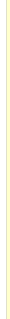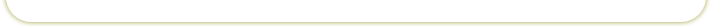Department of Land, Air and Water Resources - University of California, Davis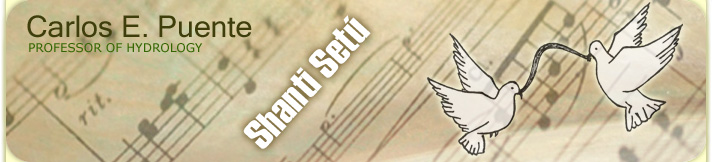Phone: +1 (530) 902-6030   Email: cepuente@ucdavis.edu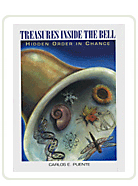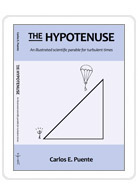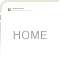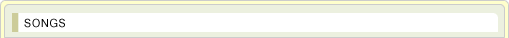### Y = XO pure geometry!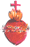Click the heart to watch the video
 Y = X is justice that illuminates, is balance that fascinates: Y = X. Y = X is the incarnate alliance, is the established reliance: Y = X. Y = X is true word that matures, is a spiral that endures: Y = X. Y = X is the precious resting place, is the state of mighty grace: Y = X. Y = X is smoothness that esteems, is a hummingbird that gleams: Y = X. Y = X is the short and precious root, is the weaving of the truth: Y = X. Y = X is a future that forgives, is crowned science that is: Y = X. Y = X is the ever tender tune, is the impartial tribune: Y = X. Y = X is all innocence that heeds, is a garden with no weeds: Y = X. Y = X is the simple clear sign, is the majestic design: Y = X. Y = X is independence that heals, is matrimony that shields: Y = X. ------------------------------ Links: From science to mercy Turbulence and the Bible More lessons from complexity Y = X is the real chaste embrace, is the goodness of a yes: Y = X. Y = X is a smile that edifies, is a spin that rectifies: Y = X. Y = X is all gentleness in us, is the everlasting plus: Y = X. Y = X is inspiration that calls, is growing to be small: Y = X. Y = X is the forgotten territory, is the improbable story: Y = X. Y = X is revelation that nests, is surrendering the rest: Y = X. Y = X is the dustless short incline, is the faithful narrow line: Y = X. Y = X is renouncing all spears, is experiencing no fears: Y = X. Y = X is the perennial giveaway, is pure life with no decay: Y = X. Y = X is the only perfect remedy, is loving, even the enemy: Y = X. (May 2002)return to top